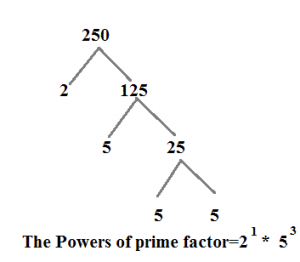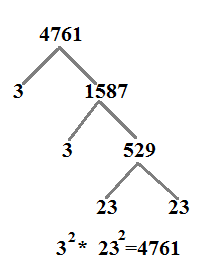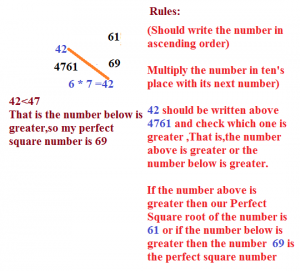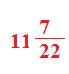# HOW TO FIND SQUARE ROOT OF ANY NUMBER-LEARN SERIES

Hi Bankersdaily Aspirants,

Learning is a step by step process and you should gradually progress in that to ace the process. As you all know we have started a new Learn Series to help with your preparations for various exams like IBPS PO , IBPS RRB Scale I officer , IBPS RRB Office Assistant , IBPS Clerk , IBPS SO , SBI PO , SBI Clerk , SBI SO and many other exams in this type. Nevertheless this series will also contribute you all the tips and tricks to every miscellaneous sections and also all the important topics which are asked in the exams. If you haven’t looked at the Learn series , Please Click the below link to learn the new concepts.

### INTRODUCTION:

Square root  of a number is not asked separately in Competitive exams but it is asked in Simplification topic,in which we can score 5 marks in less than 5 minute.Before getting into this topic,let us look in how to square and cube a number,

Now,let us look,how to find square root of a number,

### HOW TO FIND SQUARE ROOT OF A NUMBER:

Whenever a number is given to take square root there is 2 steps involved

Step 1:Is to check whether the given number is perfect square number or not

Step 2:Finding Square Root of given number

### STEP 1:

Whenever a number is given to take square root,just see whether a given number is perfect square number or not,

There is 3 condidtion,

Condition 1:

The given number should not end with 2,3,7,8….

Example:

523,628 These numbers end with 2 & 8 therefore this is not a perfect square number.

Condition 2:

The sum of digits of a given number should be 1,4,7,9

625=6+2+5=13=1+3=4

Therefore the number is perfect square number

Condition 3:

The number should be prime factor which should have powers as 2,4

Example:

1)250Here the Powers of prime factor are not 2 & 4,therefore this number is not a Perfect Square number.If you become well verse in finding the square root of a number then you will say whether the number is perfect square number or not by just looking into the number itself.

Given number should satisfy all the above mentioned 3 conditions to be a perfect square number.

1)Find the Square root of 4761.

### TO CHECK WHETHER THE GIVEN NUMBER IS PERFECT SQUARE NUMBER OR NOT:

Condition 1:

The number should not end with 2,3,7 & 8

Our number ends with 1 So,It satisfies 1st condition.

Condition 2:

The sum of digits of a given number should be 1,4,7 & 9

My Number is 4761=4+7+6+1=18=1+8=9

Condition 3:

The Power of Prime Factor may 2,4

4761It’s Satisfies the above three Condition

### FINDING UNIT DIGIT:

Take Unit Digit of any given number  ends with 1,4,5,6,9,0…

Note:

1)If given number ends with 1 then the unit digit of number may be 1,9

2)If given number ends with 4 then the unit digit of a number may be 2,8

3)If given number ends with 5 then the unit digit of a number may be 5

4)If given number ends with 6 then the unit digit of a number may be 4,6

5)If given number ends with 9 then the unit digit of a number may be 3,7

6)If given number ends with 0 then the unit digit of a number may be 0

In our Example:

4761

The Last number ends with 1,So the unit digit of the number may be 1 or 9

### FINDING TEN’S DIGIT:

Since we are going to find square root of a number,Leave the last 2 digits of the given number and take the remaining number & Check which is nearest perfect square number

In our Example:

4761

Leave the last two digit,then Remaining number is 47

Nearest Perfect Square number to 47 is 36 which is perfect square of 6

So,the number may be Either 61  or  69

### FINDING EXACT SQUARE ROOT:

Now to identify which number is Perfect square,√4761=69

Example:√128

### STEP 1:

Whenever the number is given,Consider the nearest Perfect Square number

Eg:128 Nearest Perfect Square =121=11²

### STEP 2:

1)Subtract the given number with the Perfect Square =128 -121=7

2)Then the format for writing the Square Root of a number

=Nearest Square (Difference / 2* Nearest Square )

=11 (7/2*11)## RELATED ARTICLES:

FEDERAL BANK PO & CLERK 2017 NOTIFICATION RELEASED – APPLY ONLINE

TIPS AND TRICKS TO CRACK REASONING SECTION IN IBPS PO PRELIMS 2017

PIPES AND CISTERN PART 3-LEARN SERIES